## The QPath Blog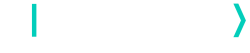# Designing Complex Circuits with QuantumPath®

AuthorsOne of the objectives of QuantumPath® is to provide tools that make the design of quantum circuits more practical and efficient for users. To this end, the tool known as Q xCompositor, a new QuantumPath® tool developed in Microsoft Excel , was introduced in the Q Assets Compositor® family . Q xCompositor allows for the creation of different gate circuits in a simpler and scalable way.

An example of its usefulness can be found in The QPath® Blog , where a 100-qubit QRNG (Quantum Random Number Generator) is implemented. The article shows how Q xCompositor facilitates the handling of large numbers of qubits by making use of the full integration of Excel and QPath® features. Based on this knowledge, the current article shows the convenience and advantages of using the Q xCompositor tool to work with circuits of great depth, or with those containing certain design patterns. For this purpose, it is proposed as an example a quantum solver implementation of the Boolean satisfiability problem, or quantum SAT solver, investigated in the paper by Shang-Wei Lin et al. .

For this reason, this publication is structured as follows: first, the SAT problem is introduced. Second, the proposal of a quantum solution is described. Next, the implementation of the exposed model is shown in Q xCompositor, making use of both the essential properties of the utility itself and the macros provided by the Excel program. Finally, the different methods of creating quantum gate circuits are compared, thus verifying the benefits and utilities of the Q xCompositor tool.

The SAT problem

The Boolean satisfiability problem consists of determining whether an interpretation that satisfies a given Boolean formula, written in conjunctive normal form (CNF) exists. That is, given a logical expression formed by a conjunction of clauses, where each clause is a disjunction of true or false literals, the aim is to find a combination of values such that the final formula is true. If such a combination exists, the formula is called satisfactory. Otherwise, it is unsatisfactory.

As an example, analyzing the expression “F1: (not a or b) and (a)” it can be easily verified that it is formed by two clauses separated by the conjunction operator and. The first clause contains two literals, separated by the disjunction operator, or: a positive one, b, and a negative one defined by the not operator, not a. On the other hand, the second clause contains only one positive literal, a. Taking into account how the different operators act, the truth table of formula F1, dependent on variables a and b, is as shown in Table 1.

 a b F1: (not a or b) and (a) F F F F T F T F F T T T

Table 1: Truth table of “F1: (not a or b) and (a)”.

As can be seen, when variables a and b are true, the logical expression F1 takes a true value and is, therefore, a satisfactory formula.

Solution to the SAT problem

Classically, the DPLL algorithm  is used to solve this type of problem. This is a backtracking algorithm which consists of assigning the true value to a literal, simplifying the formula and, recursively checking whether the logical expression is satisfactory or not.

However, since qubits exist in quantum computing with properties such as superposition or entanglement, this makes solving this problem through quantum computing considerably faster, more scalable, and more efficient. The solution proposed in the article by Shang-Wei Lin et al.  for the SAT problem consists of making use of an adaptation of Grover’s algorithm, , by defining an oracle and a diffuser as well. Based on previous research   , the following four-step method is proposed. First, the expression to be studied is analyzed, identifying how many variables, literals and clauses are used in the definition in order to determine the number of qubits required. In the second step, the oracle of Grover’s algorithm is defined. Then, a quantum version of the diffuser is determined. Finally, the state of the system is measured.

The proposed approach to handling the logical expression consists of identifying the different literals used, and their repetitions in the different clauses, in such a way as to treat each of these repetitions individually, while keeping them entangled throughout the process. In this way, the repetitions of the same variable, handled at all times as if they were independent variables, always give the same value, since they represent the same variable. Consequently, when analyzing a Boolean formula, the number of qubits required will be the sum of: one qubit for each literal used, repeated or not; one for each clause; and one to represent the final F- expression, ordered as mentioned. The initialization of these qubits consists of placing the first occurrence of each variable in the |+ state, placing an X gate in the qubits referring to the clauses, and entangling all repetitions of the same variable.

Regarding the oracle, it will consist of three differentiated parts which will be called: Ω, and Ω‑1. Ω begins by placing an X (Id) gate to all those positive (negative) literals that appear in F. Next, a CNOT or Toffoli gate,, is located in such a way that each of the literals of F are related to the clause where they appear on. In other words, as many controls are placed as literals define the clause to be studied, and the target of the gate will be placed in the qubit corresponding to the clause where they appear on. As for the second block, Ꞙ, this will serve to unify the different clauses: that is, to apply the and operator between them. For this purpose, a multi-control gate is added, where the controls will be the different clauses and the target will be the last qubit, referring to the final expression, F. Subsequently, a Z gate is added to the last qubit and the same multi-control gate of the previous step is introduced. Finally, as its name indicates, Ω‑1 applies the same gates as Ω by inverting the order of action.

It is easy to see that the oracle will depend on the logical expression to be evaluated. However, when it comes to the diffuser, the operator will have a structure which is analogous for all expressions. In order to do so, it will be necessary to disentangle the different repetitions of the literals by means of as many CNOT gates as necessary. Then, the qubits referring to the first occurrence of each literal are chosen as representative of themselves, and the diffuser is applied to them.  Applying the diffuser consists of placing an H gate and an X gate to each of the qubits; adding a multi-control gate where the target, with a Z gate, will be the last of these qubits and the controls the rest of them; placing an X gate and an H gate, analogously to the previous step; and entangling the qubits which refer to the same variables.

Finally, having the initialization of the qubits, the oracle, formed by Ω, Ꞙ and Ω‑1, and the diffuser, one proceeds to measure the state of the system, thus obtaining the expected solution. Explaining the reasoning behind the design of the algorithm is beyond the scope of this article. However, for further information on this topic, refer to the cited article, containing such explanation, by Shang-Wei Lin et al. .

As a consequence, the circuit representing the explained algorithm when the logic expression is F1 is as shown in Figure 1.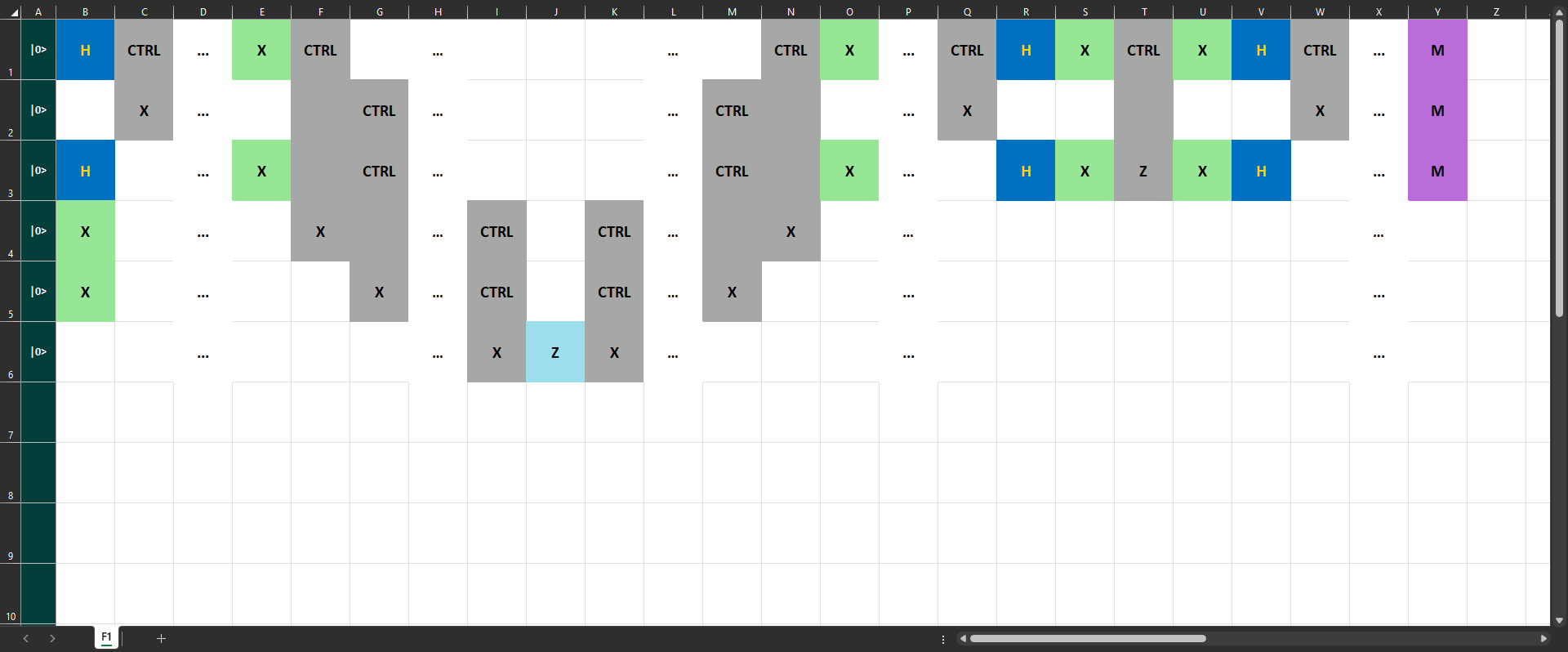Figure 1: Representation of the quantum SAT solver for “F1: (not a or b) and (a)”.

Implementation in QuantumPath® with Q xCompositor

Throughout this section it will be shown how to apply the quantum solver of the SAT problem in QuantumPath®, also called quantum SAT solver, when there is an expression somewhat more complex than F1. From Figure 1, it can be foreseen that the higher the complexity of the logical formula to be analyzed, the greater the number of qubits needed and greater the depth of the circuit. In addition, thanks to the Gate Colors option, in the xCompositor Settings tab, the colors on the different gates make it easier to visualize the symmetry of each block. Hence, instead of creating the circuit from Q Assets Compositor®, it is worth doing it from Q xCompositor due to the fact that, as mentioned before, the tool perfectly integrates both QPath® and Excel® properties.

Consequently, Q xCompositor contains the basic tools of the built-in program, such as being able to copy, paste, or even drag different parts of the circuit, tools which will be used on numerous occasions. However, at the same time, it also includes more elaborate elements such as Excel macros: automations with which repetitive tasks can be set up. The first subsection shows how to use both basic and more advanced tools simultaneously. On the other hand, the second subsection shows the option of generalizing the algorithm through macros, thus creating a complete use case where Q xCompositor tools facilitate, notably, the creation of different quantum gate circuits.

In the original article of the proposed algorithm, the following expression is used as an example: “F2: (a) and (not a or b or c) and (not c) and (a)”, which results in F2 being true if, and only if, the variables a, b and c are true. In order to provide the reader with more material, this article will work with “F3: (a or not b) and (b or not c) and (not a or b or c) and (not c) and (a)”, the truth table of which is shown in Table 2.

 a b c F3: (a or not b) and (b or not c) and (not a or b or c) and (not c) and (a) F F F F F F T F F T F F F T T F T F F F T F T F T T F T T T T F

Table 2: Truth table of “F3: (a or not b) and (b or not c) and (not a or b or c) and (not c) and (a).

In other words, F3 is a satisfactory formula, since it is true when a and b are true variables, and c is false.

To implement the circuit using Q xCompositor, it is necessary to have a gate solution created in QuantumPath®, . From there, selecting the option to create a circuit in Excel, Figure 2, the file, where the circuit will be defined, is downloaded.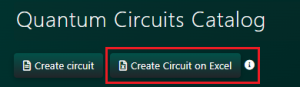Figure 2: Create circuit button in Excel.

1.     Hybrid implementation

Having the document in which the circuit will be defined, the next step is to analyze the logical expression F3 and initialize the qubits, step 1 of the algorithm. It is easy to verify that F3 is formed by three variables (a, b, and c), distributed in nine literals and five clauses. Therefore, the circuit of the quantum solver will require 15 qubits. As mentioned before, the order is sequential. Hence, the relationship between the 15 qubits and the different parameters of F3 will be as shown in Table 3.

 q1 q2 q3 q4 q5 q6 q7 q8 q9 q10 q11 q12 q13 q14 q15 a1 b1 b2 c1 a2 b3 c3 c3 a3 Cl1 Cl2 Cl3 Cl4 Cl5 F3

Table 3: Qubit mapping with F3 elements.

Where qi indicates the ith qubit; ai, bi and ci, indicate the ith occurrence of variable a, b, and c, respectively; Cli, indicates the ith clause, and F3 indicates the global expression.

In order to define these qubits, in the xCompositor Settings tab, the number 15 is entered as a parameter and, by pressing Restore Qubits, the changes are applied, Figure 3.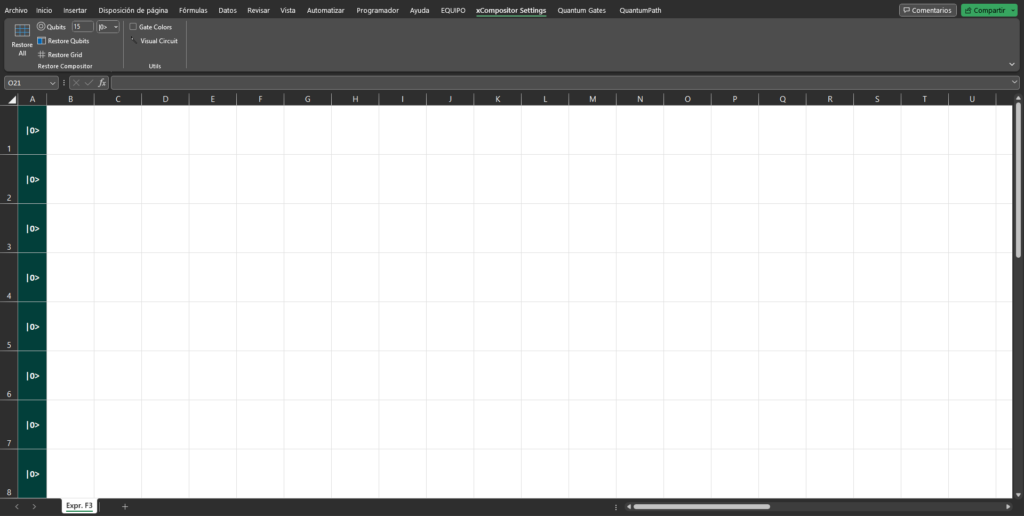Figure 3: Definition of 15 qubits with Q xCompositor.

With the purpose of initializing the qubits, the first occurrence of each variable is placed in the state |+, that is, qubits q1, q2 and q4. This is done by choosing in the drop-down, located in the first column of the document, the state in question. Next, all repetitions of the same variable are entangled, using CNOT gates. In addition, an X gate is added to every qubit referring to a clause, q10‑q14. This last step can be done by dragging down the first X door, or by inserting the doors manually. Moreover, all the gates used can be written either by selecting them in the Quantum Gates tab, or by writing them in the corresponding cells. Thus, the circuit described so far would be the one shown in Figure 4.Figure 4: Step 1. Analysis and initialization of qubits.

Following, the implementation of the oracle, made up of Ω, and Ω-1, is carried out. For this purpose, an X gate is added to each qubit representing a positive literal and the different literals are entangled with the clauses in which they are contained. At this point the Ω block, shown in Figure 5, is defined, and differentiated by the barriers.Figure 5: Step 2, Ω block. Composition of clauses.

Afterwards, the five existing clauses are entangled with the qubit referring to the general expression. Then, the Z gate is added to the latter, and the clauses are disentangled again, leaving the circuit as shown in Figure 6.Figure 6: Step 2, block. Conjunction of clauses and application of the Z gate.

It is easy to see how the block, starts and ends the same way, so one can copy the first column and paste it into the next available one. However, it can also be analyzed as an inversion of column Q ‑conjunction of clauses‑, with respect to column R, Z-gate. The usefulness of approaching it this way can be seen more easily in the next block.

The implementation of the last block of the oracle, Ω-1, consists of applying all the gates performed in the Ω block in an inverted form. As can be seen from the previous step, and from the structure of the diffuser, the process of inverting certain columns is recursive in the design of the algorithm. As a result, Excel macros can be used to automate the gate inversion process. The purpose behind using macros is both to save time in defining the circuit and to make use of the many tools available to the user by integrating QPath® with Excel. Therefore, this article now explains how to create a macro that, given some contiguous columns, inverts the order of those, placing them in the given column, onward.

Due to the fact that the macros defining QPath® properties in the Q xCompositor are protected ‑for obvious reasons‑, the macro in question will be defined in a new Excel, enabled so that it can be implemented against the Q xCompositor file. To do this, create an Excel auxiliary file, called, for example, Macro SAT Solver, and save it as an Excel macro-enabled workbook, as shown in Figure 7. This is particularly important. Otherwise, it will not be possible to work with the macros correctly.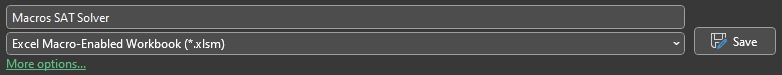Figure 7: Definition of the auxiliary Excel workbook.

Then, with both books open, in the Programmer tab of Macro SAT Solver, select Visual Basic (Alt+F11); a tool that makes use of the Visual Basic for Application, VBA, programming language. In that moment, a new window will open like the one shown in Figure 8, where the two open Excel workbooks can be seen. The elements contained in each file will also be displayed.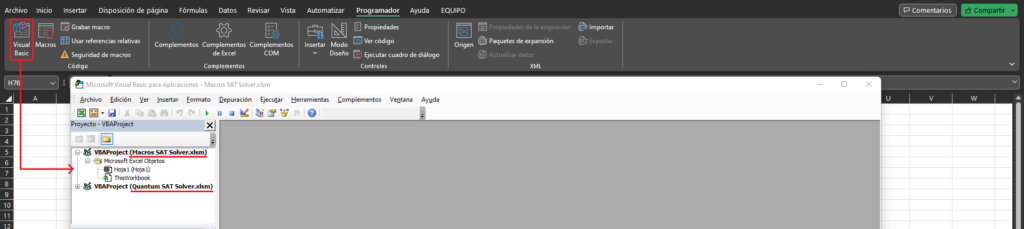Figure 8: First steps in Visual Basic.

Next, in the auxiliary workbook, a module is added, as shown in Figure 9, which will be used to write the code of the macro.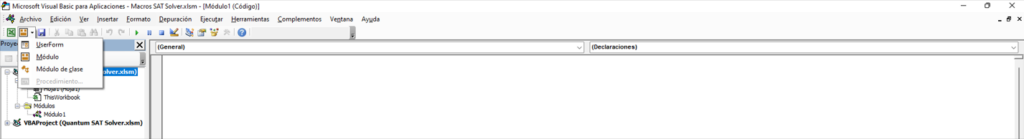Figure 9: Creation of the Module in Visual Basic.

Such code, shown in Figure 10, takes as input the set of selected columns, which must be inverted, as well as the column where the inverted columns should begin to be copied. The parameter Selection.Column takes the value of the first selected column, and Selection.Columns.Count counts the number of the selected ones. Therefore, running through the selected columns from the last one to the first one, the entire column is copied. The copied column is entered in the system, and the target column is displaced by means of the Offset command. On the other hand, since it is faster if the graphical representation of the circuit is displayed at the end, and not each time a column is copied, the ScreenUpdating command is being used.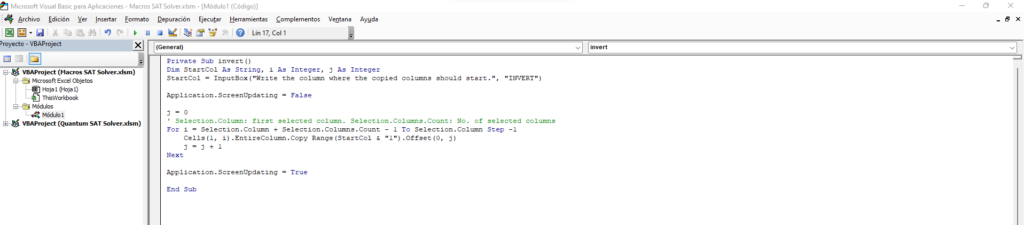Figure 10: Definition of the invert macro.

With this in place, the tools required to invert any set of gates are available in a quick and easy way. Consequently, taking into account that the block, Figure 6, belonging to the oracle of the algorithm for the expression F3, has already been implemented, now it is time to implement Ω‑1, with the macro just defined.

To do so, select in the main Excel workbook, called here Quantum SAT Solver, the area referring to the Ω block, block to invert, and run the macro. Immediately after executing it, an input box appears on the screen asking for the column where the gates are to be copied, as shown in Figure 11.Figure 11: Step 2, Ω-1 block. Execution of the macro.

Entering column U, the next available column, and selecting the OK button, the Ω‑1 block is obtained. As a result, the oracle of Grover’s algorithm with which we are working with is completely defined, Figure 12.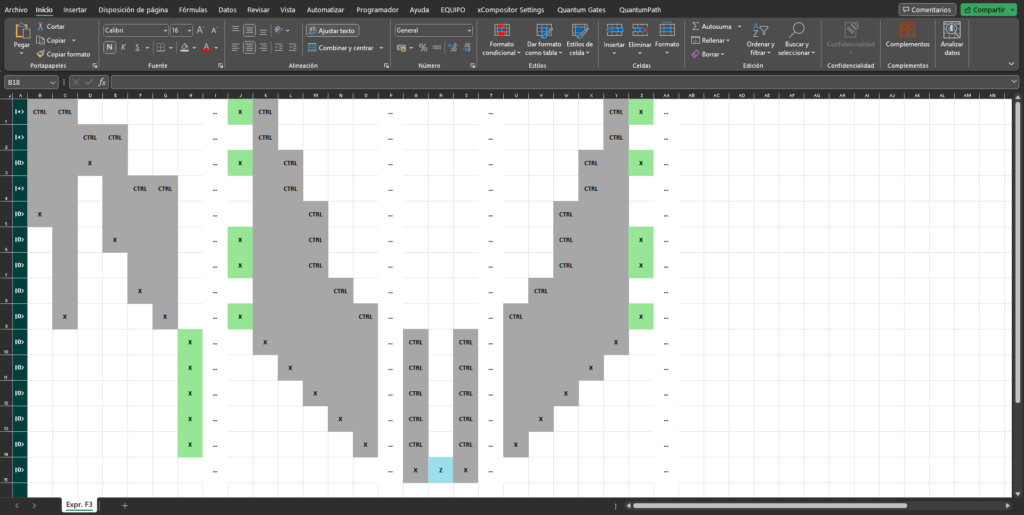Figure12: Step 2, Ω-1 block. Final description of Grover’s oracle.

The next step is to write the diffuser. As explained, the first step is to disentangle all the literals belonging to the same variables. For this step, using the macro defined, the first six columns of the circuit must be selected and placed in the next columns. Next, an H gate, and an X gate are placed on the first occurrence of each variable, that is on qubits q1, q2 and q4. The third step is to place a control gate on the first two qubits mentioned above, and a Z gate on the last one. Finally, the second and first steps of this block are repeated in reverse, for which the defined macro is particularly useful. Thus, the circuit takes the form shown in Figure 13.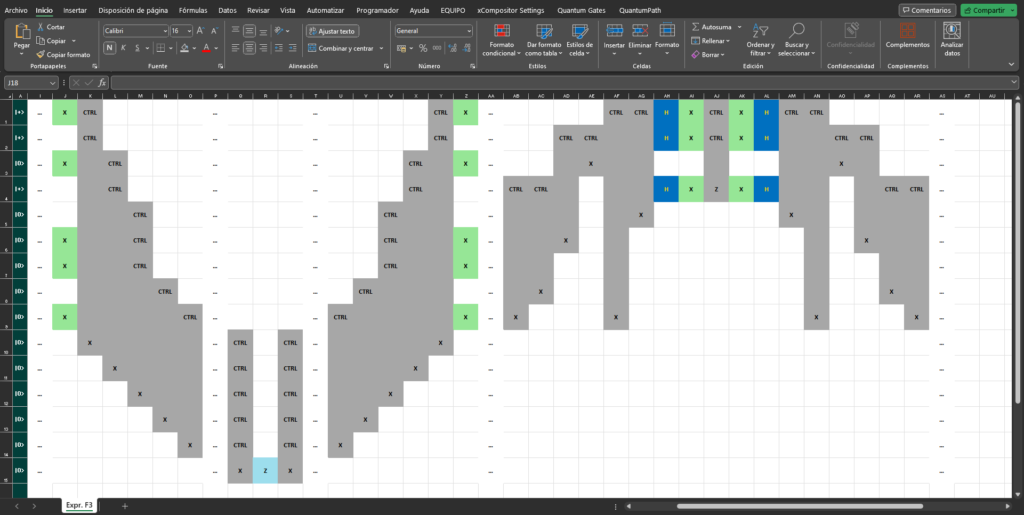Figure 13: Step 3. Integration of the diffuser.

Finally, the algorithm ends with the measurements of the different literals, as shown in Figure 14.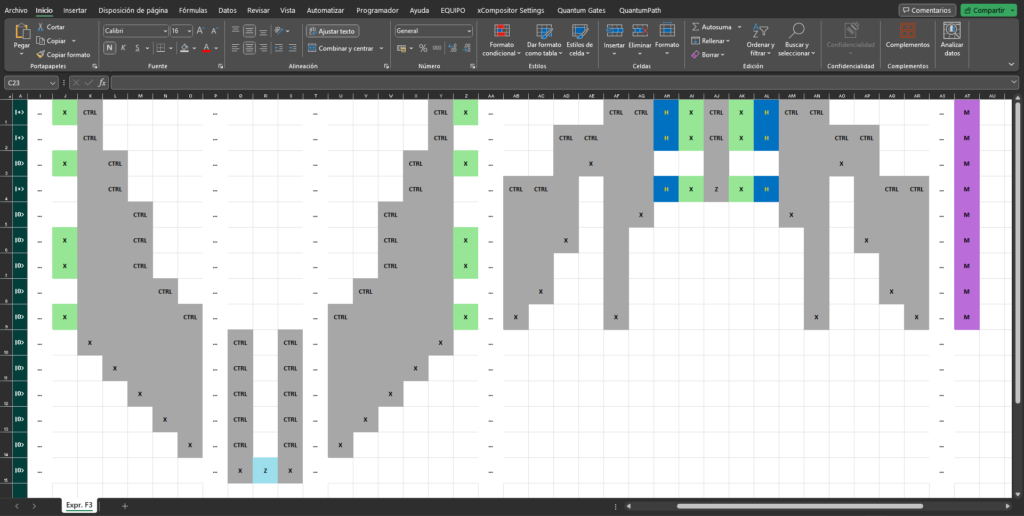Figure 14: Step 4. Measurement performance.

Notice that, due to the size of the circuit, the complete circuit cannot be displayed clearly on the screen. Therefore, it is useful to have the different colors assigned to the different gates. By visualizing the circuit in a reduced form, the colors allow the user to identify at a glance, and clearly, what type of gate each cell has, as well as the general pattern of the circuit, Figure 15.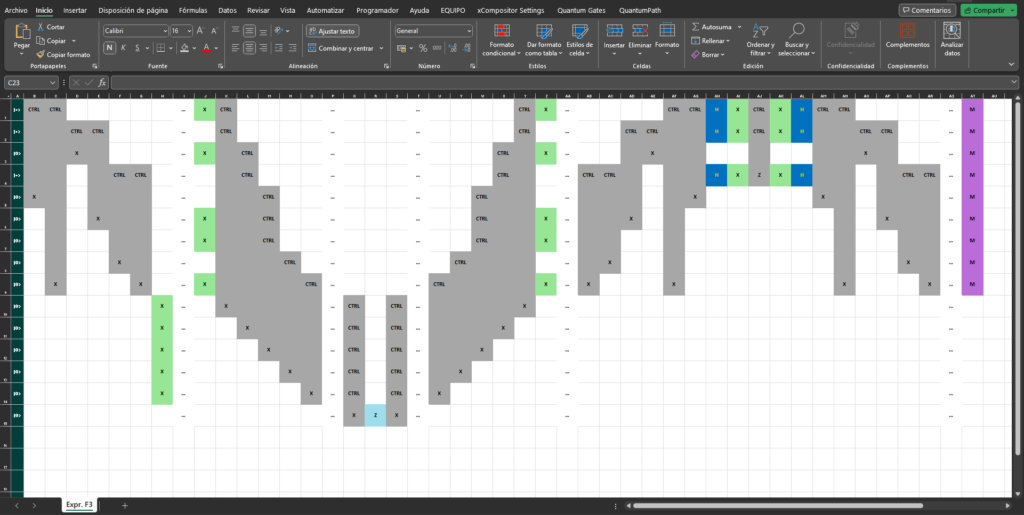Fig. 15: Representation of the quantum SAT solver. “F3: (a or not b) & (b or not c) & (not a or b or c) & (not c) & (a)”.

Having already defined the circuit corresponding to the quantum SAT solver for the expression to be analyzed, F3, all that remains is to export it to QuantumPath®, run it and analyze the results. To export it, simply select the Circuit to QuantumPath® option in the QuantumPath tab. When doing so, a window opens requesting the login and the data defining the circuit, Figure 16.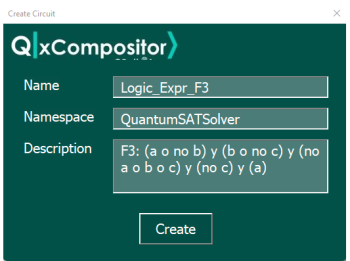Figure 16: Creation of the circuit in QuantumPath®.

In terms of execution, it is sufficient to access QuantumPath® and create the corresponding flow, which will have the structure shown in Figure 17.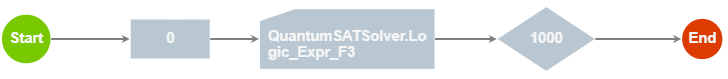Figure 17: Estructure of the flow created in QuantumPath®.

Once it has been saved, compiled and transpiled, the next step is to run it on the different machines registered in the solution being worked on. In this case, the algorithm is launched against the Amazon Braket 25qbits Local Simulator. Figure 18 shows the results obtained after running the execution.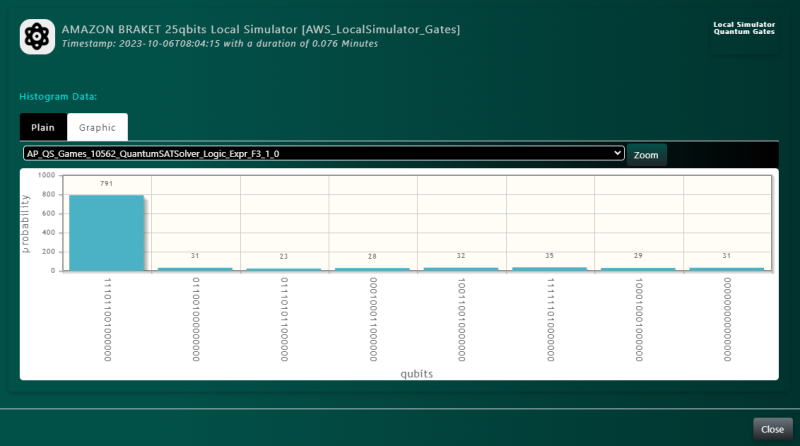Figure 18: Results obtained when running in Amazon Braket 25qbits Local Simulator from QuantumPath®.

In order to visualize these results more easily see Table 4, where the correlation between the different qubits, literals and results obtained is established. In that table it can be seen how all the qubits referring to the same variable take the same value, in this case: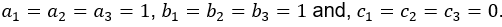this can also be seen to occur in Figure 18, where all possible outcomes satisfy this condition. This is due to the fact that, as discussed above, although all the literals are treated as independent variables, in reality they represent a single value. The correlation is achieved by the quantum property of entanglement. Hence it is particularly convenient and useful to use quantum computation to solve the SAT problem: a problem of special relevance in logic and computer science.

 q1 q2 q3 q4 q5 q6 q7 q8 q9 q10 q11 q12 q13 q14 q15 a1 b1 b2 c1 a2 b3 c3 c3 a3 Cl1 Cl2 Cl3 Cl4 Cl5 F3 1 1 1 0 1 1 0 0 1 0 0 0 0 0 0

Table 4: Qubit mapping with F3 elements and obtained results.

To conclude, comparing the results obtained, shown in Table 4, and the expected results, shown in the truth table of F3, Table 2, it can be seen how the proposed algorithm, implemented in QuantumPath®, works correctly, returning the right results for the SAT problem.

1.     Implementation with VBA

An alternative to manually creating the circuit is proposed in this section. This is done by making use of macros in order to define a single program that fully implements the algorithm. That is to say, to generalize the algorithm in order to make use of the Visual Basic tool through Q xCompositor.

To this end, and following the structure outlined in the previous subsection, the function representing the complete circuit will take as input parameters the number of clauses in a given logical expression, as well as the definition of each one of them. Next, the following steps must be performed: first, the input data is parsed. Then, the system is initialized and the blocks Ω, and Ω‑1, which correspond to the oracle, are implemented. Next, the blocks corresponding to the diffuser and the measurements are defined. Finally, the system is represented, thus obtaining the corresponding circuit.

Using VBA, the data will be entered into the system through a UserForm as shown in Figure 19. Selecting the BUILD button, the program is executed.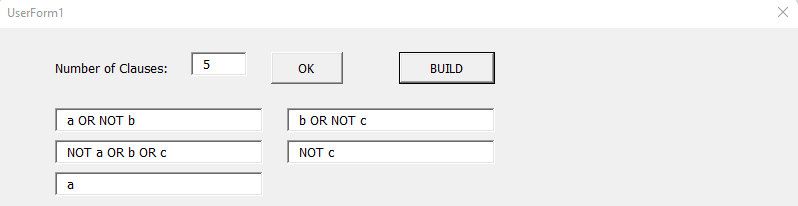Figure 19: Window with the input box and F3 parameters.

For this purpose, the core parts will be: the definition of the variables, of the subfunctions corresponding to the different blocks of the algorithm, and the visualization of the circuit. These subfunctions, described above, are shown in Figure 20.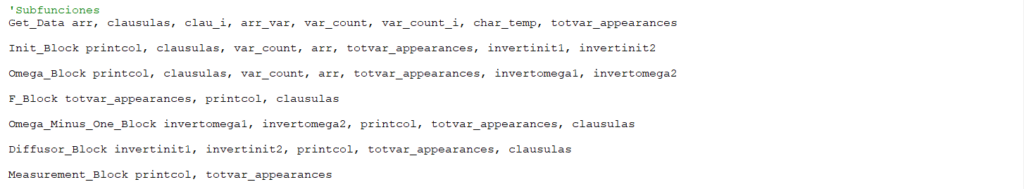Figure 20: Description of the algorithm through the main subfunctions.

Thus, as a result of the execution, the macro fills the Excel document with the circuit corresponding to the entered expression. The complete code can be obtained from . As can be seen in Figure 21, this implementation also makes use of the possibility of initializing the qubits, an advantage offered by QuantumPath®.Figure 21: Representation of the quantum SAT solver for F3, provided by VBA.

As expected, Figure 15 and Figure 21 are analogous since both correspond to the representation of the quantum SAT solver for the defined F3 expression. However, unlike the process leading to Figure 15, the technique used in this subsection allows the corresponding circuit to be generated automatically by launching the same macro. This is very useful if the algorithm is to be implemented on different logical expressions because, once the macro is written, it would only be necessary to input the expression. On the other hand, having the circuit outlined, implementing it in QuantumPath® is really simple, and should be done as shown in the previous subsection.

Conclusions

All in all, due to the relevance of the SAT problem, both in logic and in computer science, the quantization of the solution to the problem constitutes a truly significant and current use case. Such quantization implies the creation of a circuit, with certain design patterns, which grows in number of qubits and depth as the difficulty of the logical expression to be analyzed increases. It is for these reasons that the Q xCompositor tool, provided by QuantumPath®, becomes the appropriate instrument to create the corresponding gate circuit.

Q xCompositor equips the user with the circuit creation tools enabled by QPath®, at the same time as it provides the commands and utilities defined in the Excel program. As shown in the section Implementation in QuantumPath® with Q xCompositor, merging and incorporating the two elements enables the user to copy, paste, or even create macros. The usefulness of these properties is shown in the Hybrid Implementation subsection, where the advantages and facilities presented by Q xCompositor are demonstrated, as well as the need for them if a large circuit is to be defined in an agile way. On the other hand, the subsection Implementation with VBA shows the wide range of performance that can be accomplished by the different elements that make up the Q xCompositor tool, as well as the large number of actions that can be defined with the elements made available to the user.

Finally, concerning the algorithm proposed by Shang-Wei Lin et al. , implemented in this paper, a note must be made: while it is true that the execution of the algorithm with the expression “F3: (a or not b) and (b or not c) and (not a or b or c) and (not c) and (a)” returns the correct results, see Table 2 and Table 4, the reality is that the algorithm is only applicable when the truth table of the expression to be analyzed contains only one true combination. The rationale behind this, as well as the modifications needed to generalize the algorithm are beyond the scope of this research. However, the reader is invited to implement and access the resources provided in this publication and analyze them.

 J.L. Hevia, M. Piattini and G. Peterssen. QuantumPath®’s Q Assets Compositor® family is growing: tools for enterprise quantum software development. The QPath Blog. July 2023.

 Microsoft Office and Microsoft Excel are the property of Microsoft Corporation, to which Microsoft owns all copyrights.

 J.L. Hevia and A. Martin-Toledano. Q xCompositor, practical examples of quantum gate circuit development. The QPath Blog. July 2023.

 S-W. Lin, T-F. Wang, Y-R. Chen, Z. Hou, D. Sanán, and Y.S. Teo. A Parallel and Distributed Quantum SAT Solver Based on Entanglement and Quantum Teleportation.  August 2023.

 M. Davis, G Logemann, and D. Loveland. A machine program for theorem‑proving. Communications of the ACM. July 1962.

 L.K. Grover. A fast quantum mechanical algorithm for database search. Proceedings of the 28th annual ACM symposium on Theory of Computing. July 1996.

 I. Dutra and D. Fernandes. Using Grover’s search quantum algorithm to solve Boolean satisfiability problems, part 1. XRDS: Crossroads, The ACM Magazine for Students. September 2019.

 I. Dutra, D. Fernandes, and C. Silva. Using Grover’s search quantum algorithm to solve Boolean satisfiability problems, part 2. XRDS: Crossroads, The ACM Magazine for Students. November 2019.

 Quantum software development platform. QuantumPath®. 2020

 Quantum SAT solver macro. 2023.